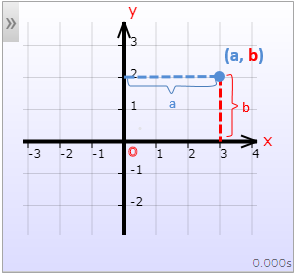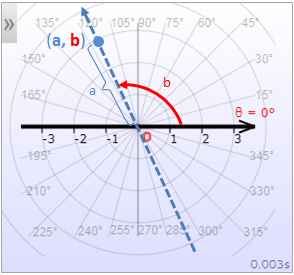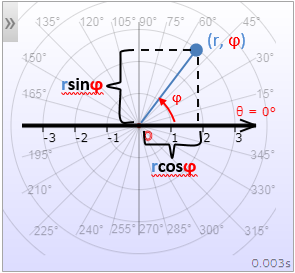# Cartesian & Polar Coordinate Systems

In this article we describe the two types of coordinate systems that are used in a plane, namely, the Cartesian and the polar coordinate systems that this graphing calculator utilizes.

Just as a number line or axis (one-dimensional coordinate system ) is used to locate a real number on a line, a coordinate plane or a two-dimensional coordinate system is used to locate an ordered pair (a, b) in a plane. There are two types of coordinate systems which are used in a plane, namely, the Cartesian coordinate system and the polar coordinate system.

The Cartesian coordinate system is formed by two intersecting axes with the common origin. It's usual to draw the axis that contains the values of the first coordinate horizontally and the other vertically. It's also common to denote them by letters x and y and call them the x-axis and the y-axis, respectively.

The polar coordinate system uses one reference axis, usually horizontal, which is called the polar axis, whose origin is called the pole. Instead of a second axis, polar coordinate system uses angles which are measured from the polar axis about the pole.

The following figures shows the coordinate systems used in a plane.

Either of the Cartesian coordinate system or polar coordinate system can be used to locate or plot points and graph functions, graph equations, graph parametric curves. The following figures illustrate how the point (a, b) is plotted in each coordinate system for a = 3 and b = 2.Fig 3. Cartesian plot: a = 3, b = 2Fig 4. Polar plot: a = 3, b = 2 (≃ 115°)

In the Cartesian coordinate system with the x-axis and y-axis as described above, the first coordinate, a, is called the x-coordinate (or abscissa) and the second coordinate, b, is called the y-coordinate (or ordinate).

When using the polar coordinate system, a is called the radial coordinate and b is called the angular coordinate or (polar angle). The radial coordinate is the signed distance from the pole and the angular coordinate is the angle by which the polar axis has to be rotated to locate the point (a, b) in the polar coordinate system.

The point (a, b) can be described as x = a and y = b in the Cartesian coordinate system. In the polar coordinate system, it is customary to use r and θ (or φ) and write r = a and θ = b (or φ = b).

Although every ordered pair (a, b) has a unique location in a coordinate plain, a location (point) in the polar coordinate system can have more than one polar representations because adding multiple of 2π (or 360°) to an angle does not affect the "location" of that angle. So, the ordered pairs (a, b+2π) determines the same point as (a, b) in the polar coordinate plain. Also, when you add π to the angular coordinate, changing the radial coordinate a to -a determines the same point as (a, b) as the following illustrations show.

To summarize, all the ordered pairs (a, b), (a, b+2nπ) and (-a, b+(2n+1)π) identify the same point in the polar coordinate system for the integers n.

If the axes are perpendicular, as seen above, the Cartesian coordinate system in a plane is called the rectangular coordinate system or orthogonal coordinate system. However, the axes need not be perpendicular, in which case we have a non-orthogonal coordinate system, as the following figures demonstrate. Also note that the polar axis in the polar coordinate system need not be horizontal.

## Conversion between Polar and Cartesian Coordinates

An ordered pair (a, b) when plotted in the Cartesian coordinate system, generally speaking, has different location from when plotted in the polar coordinate system (with the same origin and pole). Given the coordinates of a point in one coordinate system, you might ask, what is the coordinates of that point in the other coordinate system so that they both locate the same point. To answer this question, we assume the in Cartesian coordinate system the independent axis is horizontal and the dependent axis is vertical and also the polar axis coincide with the x-axis and share the same origin, and that both Cartesian axes and the polar axis have the same scales.

### Converting Polar Coordinates to Cartesian Coordinates

Converting polar coordinates to Cartesian coordinates, by the way trig functions are defined on a trig circle, is straightforward. Referring to the following figure, it is obvious that the point (r, φ) in the polar coordinate system and the point (rcos(φ), rsin(φ)) in the Cartesian coordinate system coincide.Fig 10. Polar to Cartesian: x = rcos(φ), y = rsin(φ)

So we have the following formula for converting polar coordinates to Cartesian coordinates.

Polar to Cartesian Coordinates

x = r*cos(φ) y = r*sin(φ)

Note: the above conversion formula holds even if r is negative. Because if r < 0, then x = -|r|*cosφ = -(-r)*cosφ = r*cosφ and similarly, y = -|r|*sinφ = -(-r)*sinφ = r*sinφ.

### Converting Cartesian Coordinates to Polar Coordinates

As you see from the following figure regardless of the location of the point (x, y), by the Pythagorean Theorem, we have r =√( x2 + y2) and we have tan(φ) = y/x Because tan-1() always gives an "angle", between -π/2 and π/2 we have φ = tan-1 (y/x) if (x, y) is in the first or fourth quadrant (that is if x > 0) by the definition of tan-1.

Because we are dividing by x, x must be non-zero. For the case x = 0 the point is on the y-axis and the angular coordinate is θ= π/2 and the radial coordinate is r = y.

If (x, y) is in the second or third quadrant (that is if x < 0) we have to add (or subtract) π to the tan-1 (y/x) to obtain the corresponding polar angle.Fig 11. Cartesian to Polar: r = √(x2+y2), φ = tan-1(y/x)

To summarize

Cartesian to Polar Coordinates (1)

If x = 0
r = y φ = π/2
Otherwise
r = √(x2+y2)
φ = {
tan-1(y/x)      if x > 0 tan-1(y/x)+π if x < 0

Alternatively, when x < 0, instead of adding π/2 to tan-1(y/x) we can multiply r by -1 to get the corresponding radial coordinate.

Cartesian to Polar Coordinates (2)

If x = 0
r = y φ = π/2
Otherwise
r = {
√(x2+y2) if x > 0 -√(x2+y2) if x < 0
φ= tan-1(y/x)

The radial coordinate in above can be expressed concisely by using the signum function, sgn(), as

Cartesian to Polar Coordinates

If x = 0
r = y φ = π/2
Otherwise
r = sgn(x)√(x2+y2) φ = tan-1(y/x)